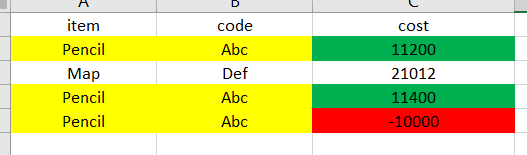# Sum if the cost value column larger than 0

Hi Everyone.

I have datatable as attached file.

I want to check if the “item” and the “code” columns are same and the value at the “cost” columns are negative or positive then it will Sum the cost value as below picture.

How to do that with linQ ?Book1.xlsx (8.2 KB)

Hi @Mr.H ,

Steps to be followed for the finding sum of cost of products :

2. dtResult =dtTable.clone
3. dtResult=(From dte In dtTable.AsEnumerable Group dte By item=dte(0).ToString.Trim,code=dte(1).ToString.Trim Into Group Select dtResult.Rows.Add({item,code, Group.Sum(Function (x) CInt(x(“cost”).toString.Trim))})).CopyToDataTable

Happy automationThanks ,
Priyanka.

1 Like

Hi Bro.

Thanks you , but it run not exactly as my condition. I only sum if the cost value is larger than 0.

As in my picture, i only want to sum the 2 row as highlighted green.Hi,

Hope the following helps you.

``````dtResult = dt.AsEnumerable.GroupBy(Function(r) Tuple.Create(r("item").ToString,r("code").ToString)).Select(Function(g) dt.Clone.LoadDataRow({g.key.item1,g.key.item2,g.Where(Function(r) Int32.Parse(r("cost").ToString)>=0).Sum(Function(r) Int32.Parse(r("cost").ToString))},False)).CopyToDataTable

dtResult = dtResult.AsEnumerable.Concat(dt.AsEnumerable.Where(Function(r) Int32.Parse(r("cost").ToString)<=0)).CopyToDataTable
``````

Sample20211104-2.zip (8.3 KB)

Regards,

1 Like

Hi Bro.

Thanks you very much!

1 Like

This topic was automatically closed 3 days after the last reply. New replies are no longer allowed.